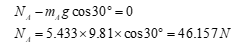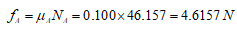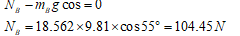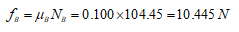Question
20 views

To apply the principle of work and energy to a system of particles.  The principle of work and energy can be extended from one particle to include a system of particles as follows:

T1+∑U1−2=∑T2

where ∑T1 is the system's initial kinetic energy, ∑U1−2 is the sum of the work done by all external and internal forces acting on the system's particles, and ∑T2 is the system's final kinetic energy. When the particles either are undergoing only translational motion or are connected by inextensible cables, the internal forces of the particles negate each other; only the external forces are required in the principle of work and energy.

When frictional forces are present, the work done by the frictional force is UF=μkNs, where μk is the coefficient of kinetic friction, N is the normal force, and s is the body's displacement. In this equation, the work done by friction represents both the external work of friction and the internal work that is converted into various forms of internal energy, such as heat.

As shown, two blocks, resting on different inclines, are connected by an inelastic cable that passes over a frictionless pulley(Figure 2). Block A weighs 12.0 lb and block B weighs 41.0 lb . The incline angles are θ1 = 30.0 degrees and θ2 = 55.0 degrees , and the coefficient of kinetic friction between the blocks and the inclines is μk = 0.100. When the blocks are released from rest, block B slides down its incline. What is the magnitude of block A's velocity, vA, after block B has slid 6.90 ft ?

check_circle

Step 1

given:

The mass of Block A = 12.0 lb

The mass of block B = 41.0 b

The inclined angles are 30.0 degree and 55.0 for A and B respectively

The coefficient of friction is 0.100

Step 2

Solution:

First, we find the friction forces for block A and B respectively :

For mass A:

The mass of block A, mA = 12.0 lb = 5.433 kgHere , NA is the normal reaction at block A.

The friction force is given as :Step 3

For mass B:

The mass of block B , mB = 41.0 lb = 18.562 NHere , NB is the normal reaction at block B.

The friction force is given as :...

### Want to see the full answer?

See Solution

#### Want to see this answer and more?

Solutions are written by subject experts who are available 24/7. Questions are typically answered within 1 hour.*

See Solution
*Response times may vary by subject and question.
Tagged in